# 写代码是一种艺术，甚于蒙娜丽莎的微笑！

 博客园  :: 首页  :: 新随笔  :: 联系 :: 订阅:: 管理

## 汉诺塔问题void hanoi(int n,char x,char y,char z){
if(n == 1)
move(x,1,z);
else{
hanoi(n-1,x,z,y);
move(x,n,z);
hanoi(n-1,y,x,z);
}
}（图片来源于“码农翻身”公众号）

hanoi(n-1,x,z,y);


move(x,n,z);

hanoi(n-1,y,x,z);

## n的阶乘问题

f(n) = n!

int factorial(int n){
if(n == 1)
return 1;
else
return n * factorial(n-1);
}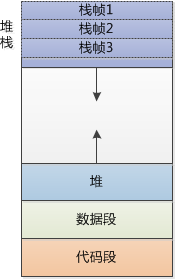（图片来源于“码农翻身”公众号）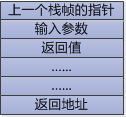（图片来源于“码农翻身”公众号）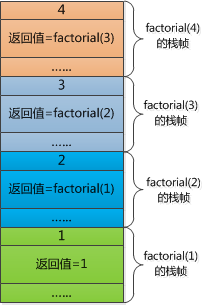（图片来源于“码农翻身”公众号）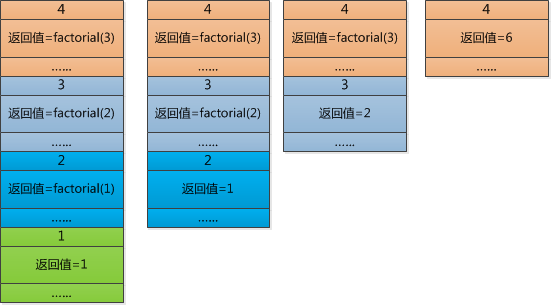（图片来源于“码农翻身”公众号）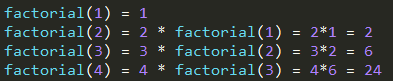int factorial(int n,int result){
if(n == 1){
return result;
}
else{
return factorial(n-1,n * result);
}
}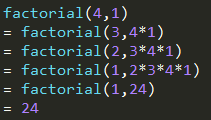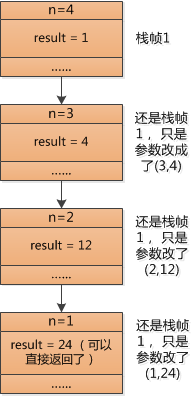（图片来源于“码农翻身”公众号）

“码农翻身” 公共号 : 由工作15年的前IBM架构师创建，分享编程和职场的经验教训。posted on 2016-11-14 22:17  RunningSnail  阅读(33561)  评论(2编辑  收藏  举报